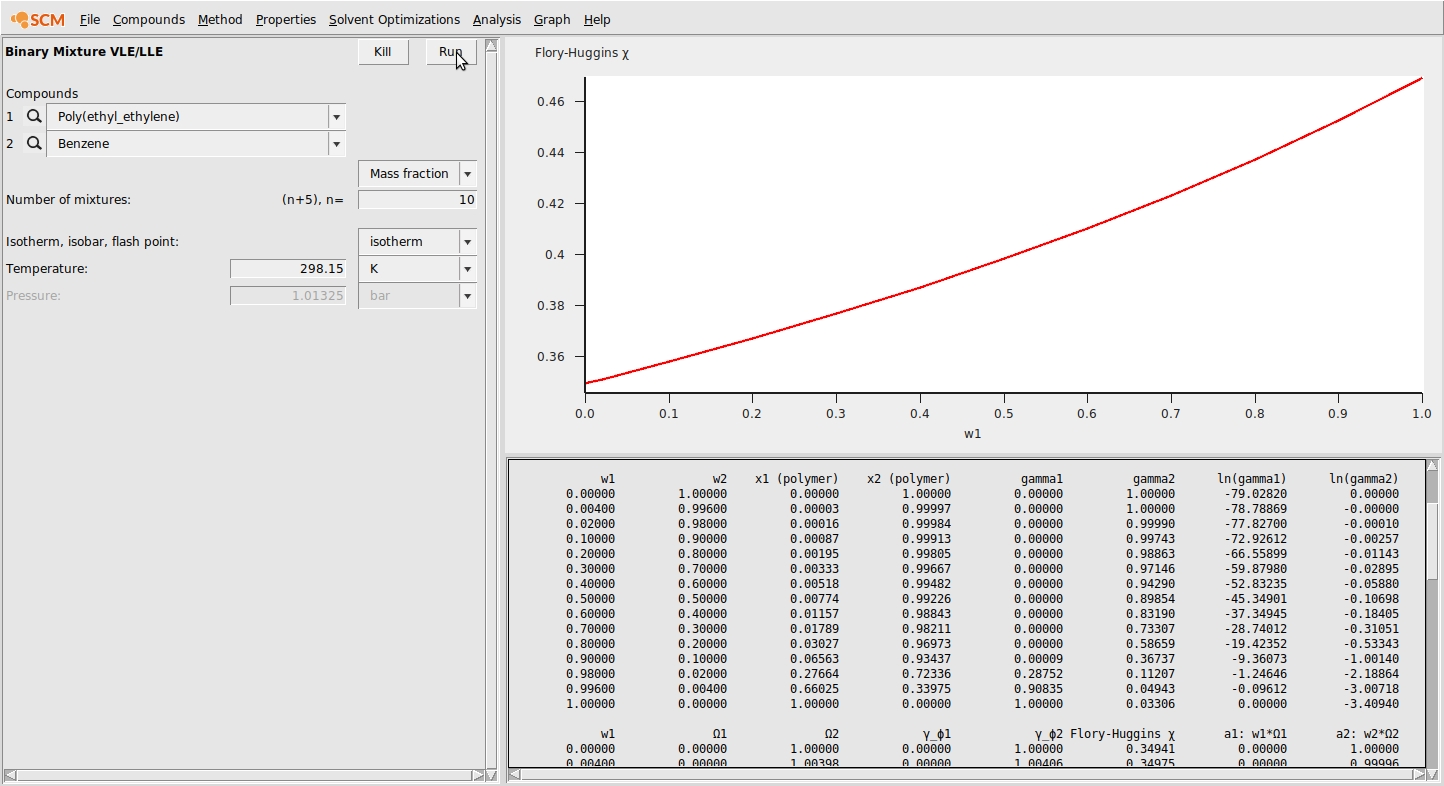# Polymers¶

The COSMO-RS program can also be used to calculate thermodynamic properties of polymers and polymer-containing mixtures. The theory and equations behind this are described in the COSMO-RS documentation. This tutorial will focus on practical details of performing calculations with polymers in the COSMO-RS GUI.

## The ADFCRS Polymer Database¶

The COSMO-RS Polymer Database contains >200 structures of common polymers, all represented as the central unit of a trimer as described in the COSMO-RS manual. Because the simplest procedure used to generate the structure of a monomer still requires the calculation of a trimer, it is recommended to use the polymer database for this tutorial as adding user-defined polymers will likely be time-consuming (minutes-days, depending on the size of the polymer).

To quickly get started with polymers in COSMO-RS, download the COSMO-RS Polymer Database. More information about the database can be found here.

## Selecting/inputting database compounds¶

After downloading and unzipping the database, open the ADFCRS GUI. To use the database in COSMO-RS, follow the steps shown below.

Select Compounds → Add Compound(s)
Navigate to the Polymer Database directory
Click on monomers.compoundlist and select Open

Note

Polymeric compounds can also be added by SMILES string. Be sure to input a polymer in CurlySMILES format. See the Polymer Database Website for examples of the CurlySMILES format. More specifically, you can see the CurlySMILES of polystyrene here or read the CurlySMILES paper

## Inputting your own polymers¶

It is also possible to input new polymers to the database and use these structures as you would with any polymer in the database. Like with all other COSMO-RS compounds, we must first perform a geometry optimization and a single point COSMO calculation. The required steps for this calculation are as follows:

Start AMSinput (SCM → New Input)
Select Task → COSMO-RS Compound
Input the structure of a single monomer unit of your polymer
Add dummy (“Xx”) atoms corresponding to where the monomer is attached to other monomers in the polymeric structure
Go to Model → Solvation
click “Generate” next to “COSKF trimer”
Save/run your input file

We will illustrate the process of new polymer input using polyethylene below:

(1) Select Task → COSMO-RS Compound, Input the monomer structure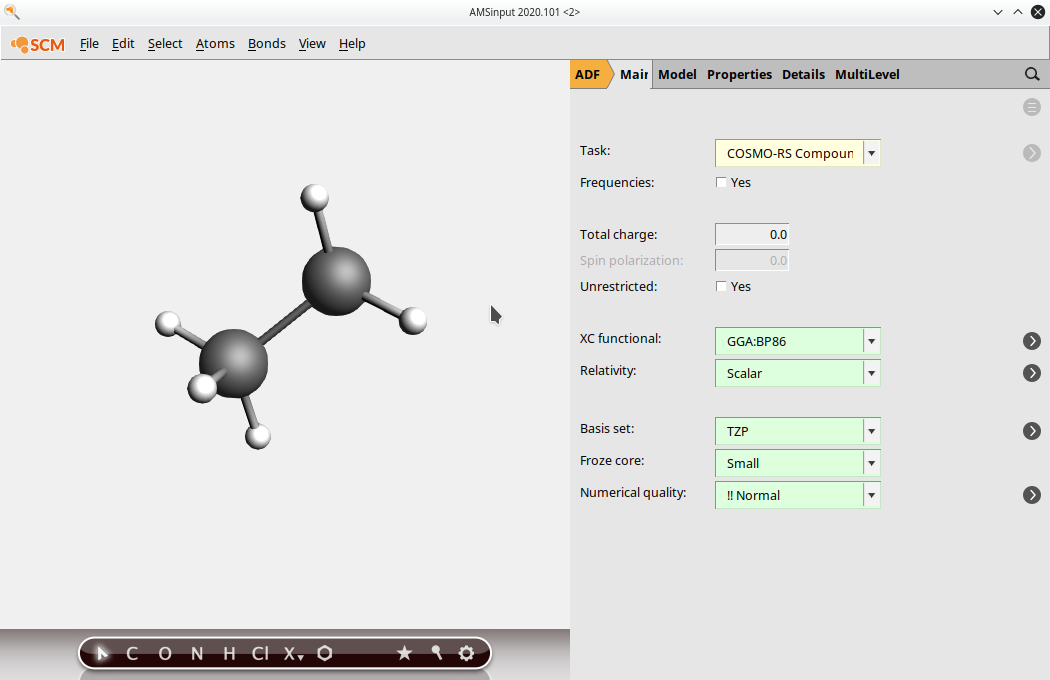(2) Select the atoms that should be replaced by other monomer units in the full polymer. Note: depending on how you have inputted the structure, you may have to add these atoms. Be sure to check that all of the correct atoms are present. Select multiple atoms by holding the Shift key and clicking.(3) Click on Atoms → Change Atom Type and replace the atoms with “Xx”(4) Go to Model → Solvation , Select Solvation Method → COSMO, Select **COSMO Solvent → CRS, and click “Generate” next to “COSKF trimer”(4.5) (Optional, but recommended) Go to the DFTB menu, select GFN1-xTB in the Model field, and press the Pre-optimize button. This will provide a good initial geometry for the subsequent ADF calculation.(5) Go back to the ADF panel and select Task: COSMO-RS Compound save your input file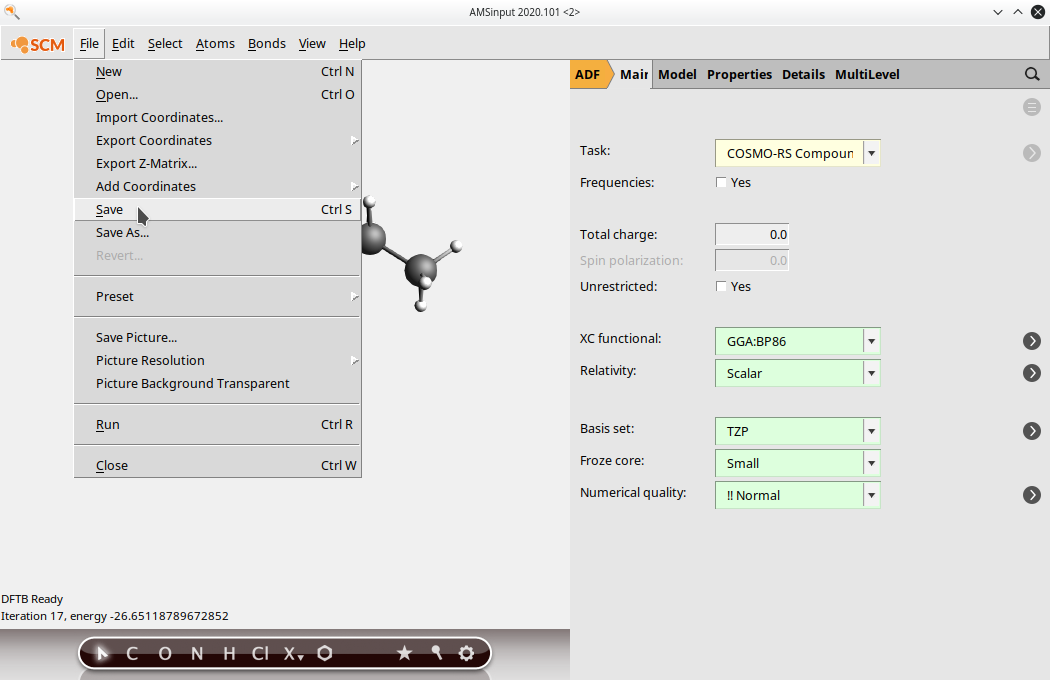(6) Run the job (File → Run). After running the job, open the .coskf file in the COSMO-RS GUI. You can see the COSMO surface by first selecting your new compound on the Compounds → List of added compounds window. Then press the “Show 3D” button. From AMSview, click Add → COSMO: Surface Charge Density → On COSMO surface points. This should produce the image below: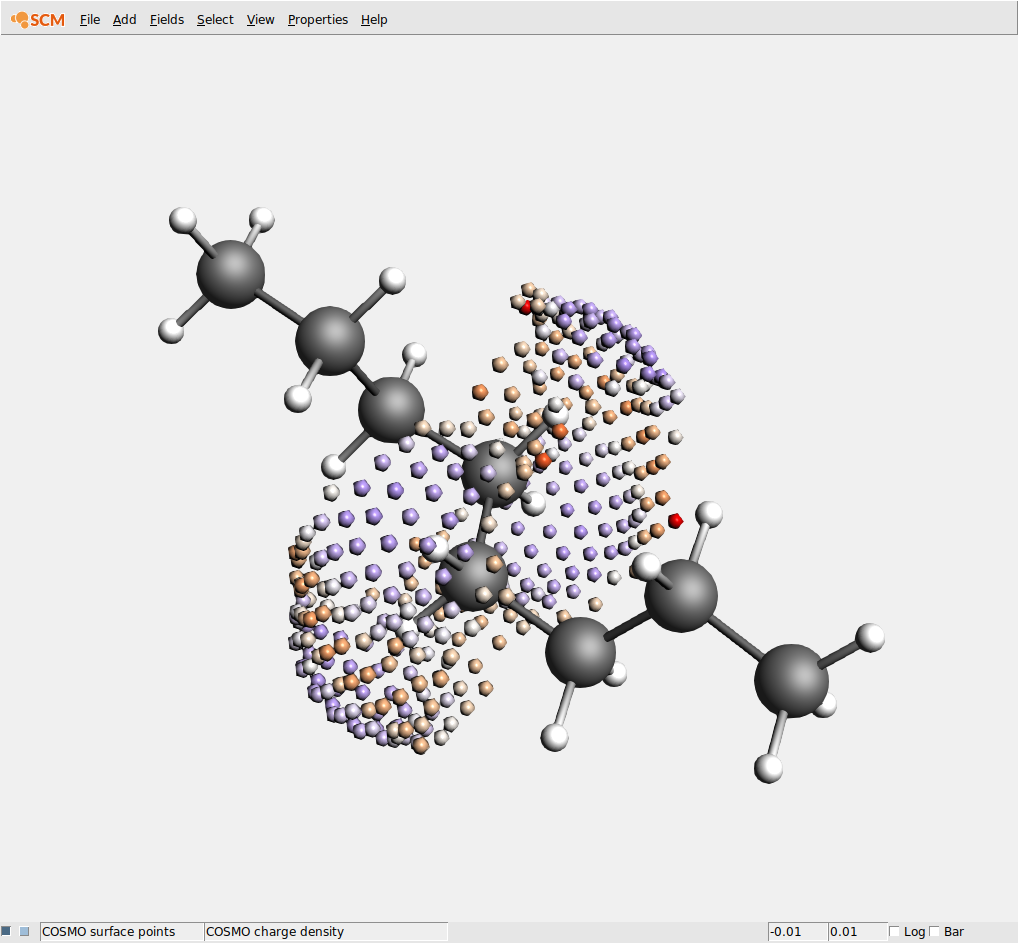## Inputting necessary property values¶

### Polymer/Average Molecular Weight¶

Because polymers of the same type can come in many lengths, it is important to be able to adjust the average molecular weight of a polymer. This is done from the compounds menu: Compounds → List of Added Compounds. Below, we show an example of setting the average molecular weight for Poly(ether_ether_sulfone) (PEES):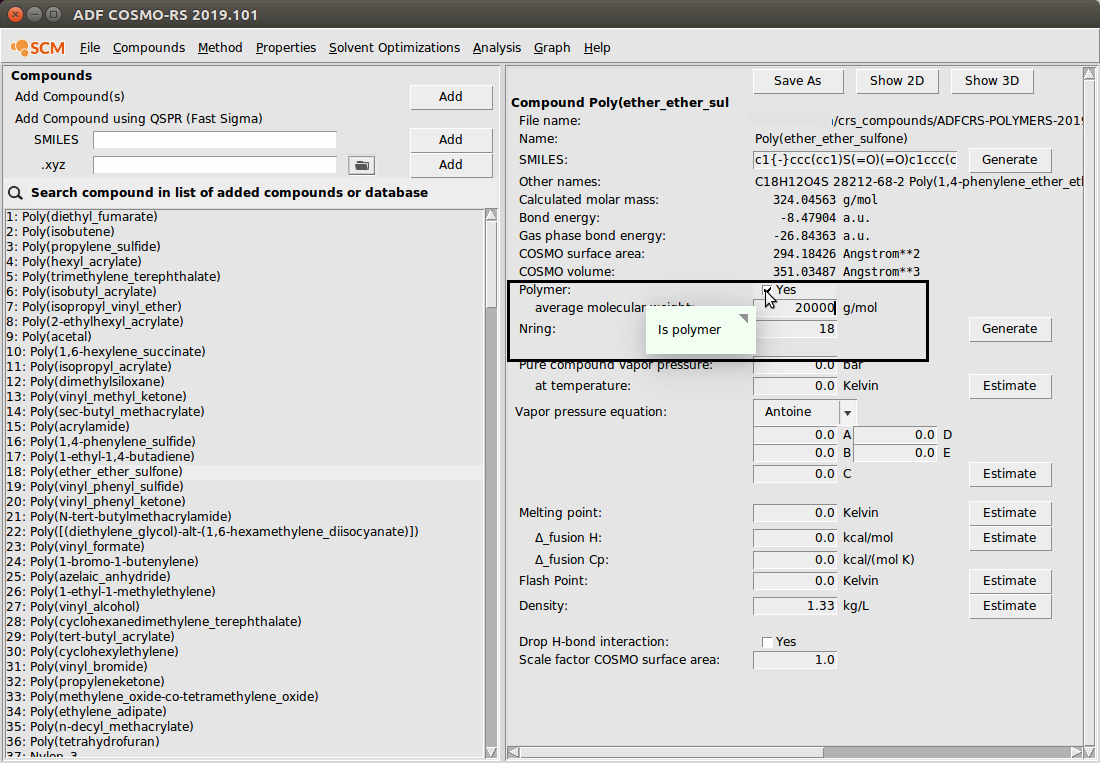Additionally, to specify that a compound should be treated like a polymer, the Polymer box should be checked (shown in the image above). The number of repeat units will be set to the average molecular weight divided by the molar mass of the compound (the compound is assumed to the be monomer).

### Density¶

Due to a modified combinatorial term for polymers, densities are required for every species in a calculation involving any polymers. In the ADFCRS database, many polymers already have a recommended density value, which should provide reasonable results under standard temperature and pressure conditions. However, it is well-known that for many polymers these density values can be sensitive to temperature and solvent. In these cases – or in cases where there is no recommended density value given – the density can be input from the compounds menu: Compounds → List of Added Compounds.

Here, we show an example of inputting the density for Poly(ether_ether_sulfone) (PEES):Note

Many polymers in the Polymer Database already have a given experimental density value. This value is automatically used for the calculation if the user does not input a different value for density. These experimental values come from the the Polymer Database Website.

## Example polymer calculations¶

Polymers can be used just like other compounds in COSMO-RS/-SAC: they are simply selected as pure components or components of a mixture. If any polymers are present in the system (as indicated by the Polymer flag on the List of Added Compounds menu), then a number of special properties will be calculated. Because polymer calculations are done just like calculations for small molecules, this tutorial will cover a few example calculations without going into the details of every possible type of calculation. For a comprehensive tutorial about different problem types with the COSMO-RS GUI, we redirect the reader here.

### Activity coefficients¶

For this calculation, we estimate the activity coefficients in a Poly(ethylene)/Benzene mixture. Because this is a calculation involving polymers, we will need the density of both Poly(ethylene) and Benzene. There is a recommended value of Poly(ethylene) given (0.852), so we can use that value. For Benzene, we’ll enter a value of 0.876. Use the following summary of steps to perform the calculation:

Compounds → List of Added Compounds
Select Benzene
Set Density to 0.876
Properties → Activity Coefficients
Select 2 Components
Select Mass fraction
Select Poly(ethylene) and Benzene
Set mass fraction of Poly(ethylene) to 0.5
Set mass fraction of Benzene to 0.5
Click Run

The result of this calculation is the following:Notice that some non-standard properties are given: weight fraction activity coefficient (= mass fraction activity coefficient), volume fraction activity coefficient, and Flory-Huggins $$\chi$$.

### Vapor pressure of a mixture¶

Now, we will estimate the vapor pressure of a mixture of Poly(dimethylsiloxane), Methanol, and n-Hexane. Use the following summary of steps to perform the calculation:

Compounds → List of Added Compounds
Select Methanol
Set Density to 0.792
Click Estimate next to Vapor pressure equation
Select Hexane
Set Density to 0.655
Click Estimate next to Vapor pressure equation
Properties → Vapor pressure mixture
Select 3 Components
Select Mass fraction
Select Poly(dimethylsiloxane), Methanol, and Hexane
Set mass fraction of Poly(dimethylsiloxane) to 0.5
Set mass fraction of Methanol to 0.25
Set mass fraction of Hexane to 0.25
Adjust the temperature range from 298.15 K to 398.15 K
Click Run

The result of this calculation is the following: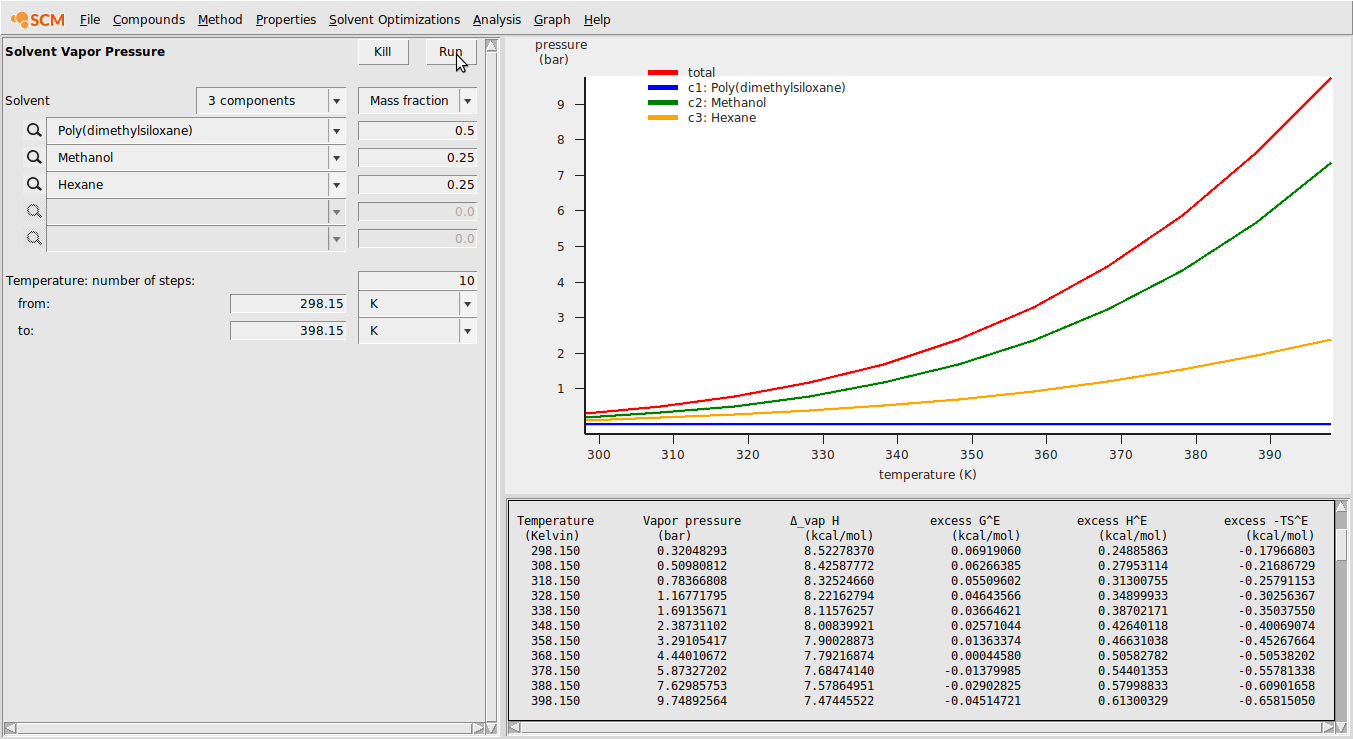### Partition Coefficients (LogP)¶

Now, we’ll calculate the partition coefficient of Methanol between a water and poly(ethylene) phase.

Compounds → List of Added Compounds
Select Methanol
Set Density to 0.792
Select Water
Set Density to 1.0
Properties → Partition Coefficients (LogP)
User Defined 2 components
Select Poly(ethylene) and Water
Set mole fraction Poly(ethylene) to 1.0 in phase 1
Set mole fraction Water to 1.0 in phase 2
Select Methanol in the Solutes (infinite dilute) box
Click Run

The output of this calculation is shown below: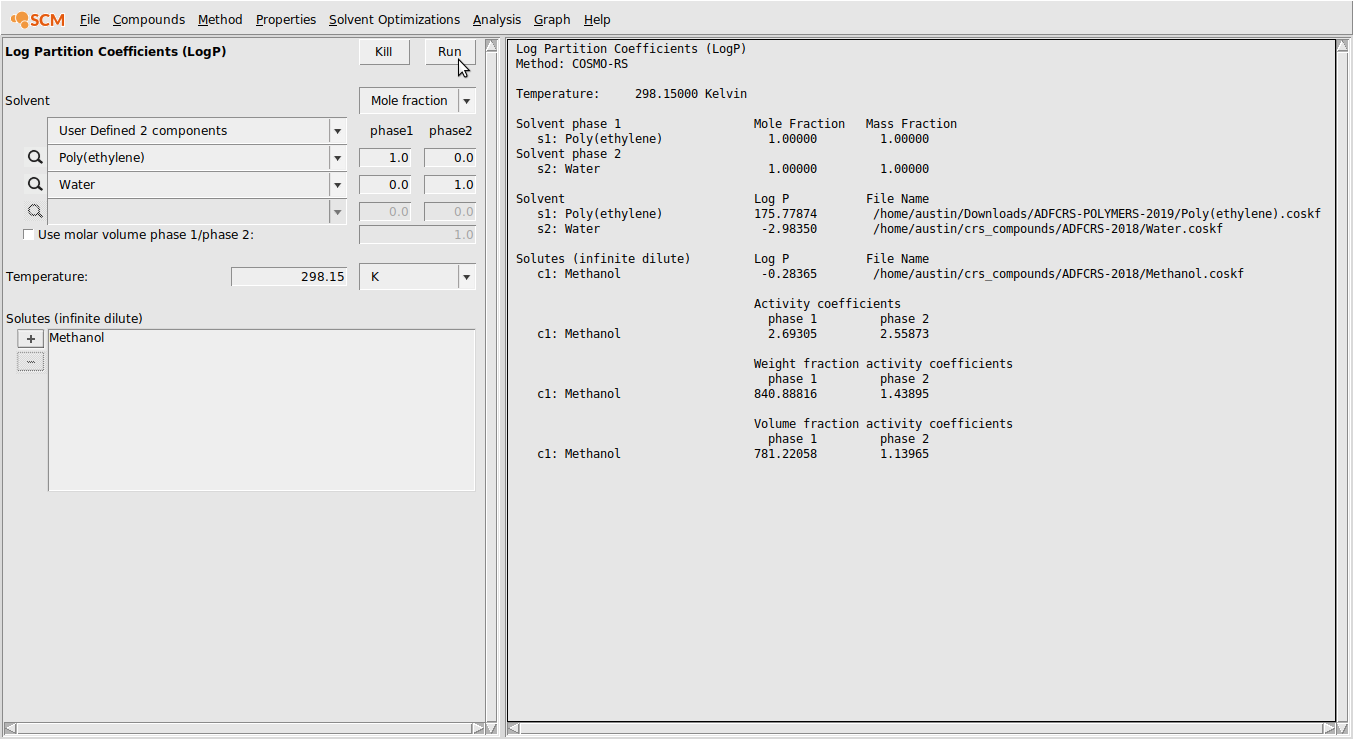### Solubility in Pure Solvents¶

In this example, we’ll calculate the solubility of Hexane gas in Poly(styrene) over the temperature range 398.15 K to 498.15 K and over the pressure range 1.0 bar to 4.0 bar.

Compounds → List of Added Compounds
Select Hexane
Set Density to 0.655
Click Estimate next to Vapor pressure equation
Properties → Solubility in Pure Solvents
Select Poly(styrene) in Pure Compound Solvents
Select Hexane as the Solute
Select Gas for the solute type
Set the temperature range from 398.15 K to 498.15 K
Set the number of steps for pressure to 3
Set the pressure range from 1.0 bar to 4.0 bar
Click Run
Click Graph → Y axes → Solubilty (g/L solvent)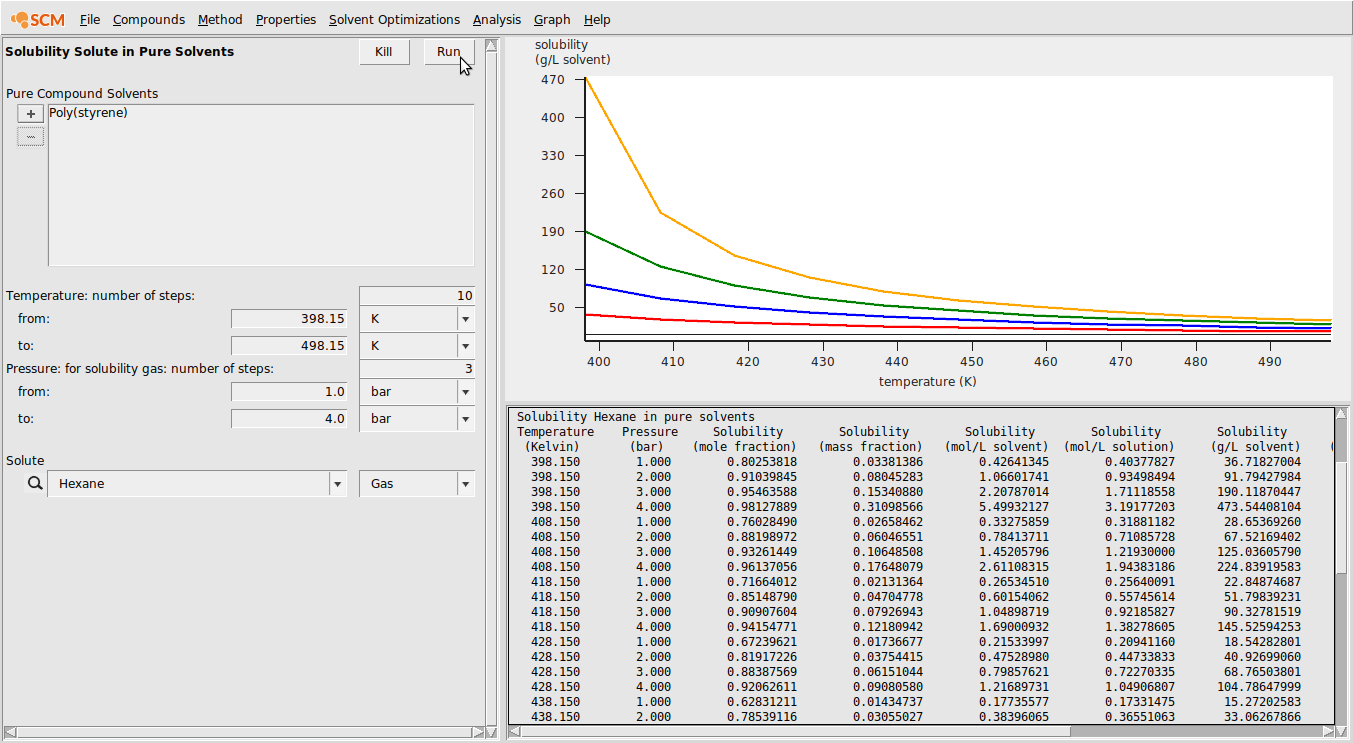### Binary Mixture and Flory-Huggins $$\chi$$¶

In this example, we will calculate binary mixture properties (including the $$\chi$$) over the entire range of compositions. We will do this for a binary mixture of Benzene and Poly(ethyl_ethylene) and use COSMO-SAC (the 2013-ADF Xiong parameters).

Compounds → List of Added Compounds
Select Benzene
Set Density to 0.876
Method → COSMO-SAC
Method → Parameters
Select the 2013-ADF Xiong parameters
Properties → Binary Mixture VLE/LLE
Select Poly(ethyl_ethylene) as Compound 1
Select Benzene as Compound 2
Select Mass Fraction
Click Run
Click Graph → X axes → w1: mass fraction 1
Click Graph → Y axes → Flory-Huggins 𝛘

The result of this calculation is the following: